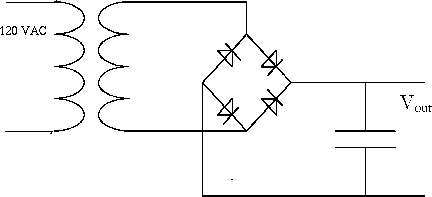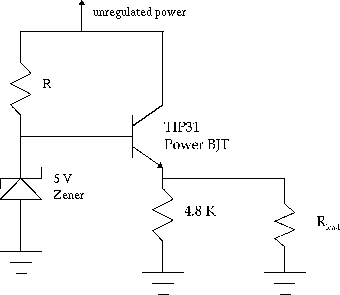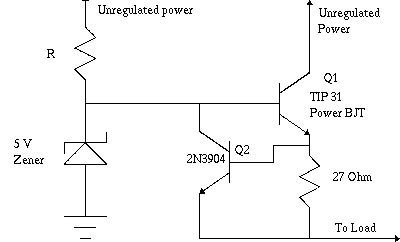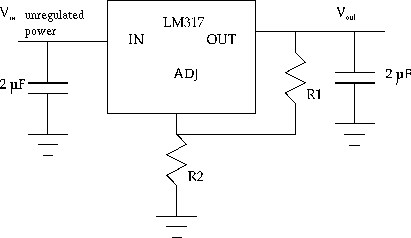EE322 Laboratory

Linear Voltage Regulators

The purpose of this lab is to investigate voltage regulation techniques for generating constant voltages and power supplies.

Pre Lab.

Full-wave rectifier.

• Calculate what the ripple will be for the full-wave rectifier both with a 1k load and with no load.
• What should the ripple frequency be?
• How will the ripple change if you double or halve the load current?

Analyze the discrete component regulator.

• Find the size of resistor that is in series with the zener (assume the unregulated voltage is 12V; during lab you will need to change this after you measure your voltage).
• Find the voltage at each point in the circuit.
• Find each current in the circuit. Do this for both loads.
• How much power is being dissipated in the load, and how much in the regulator transistor?
• How does this compare with the power rating of the transistor?
• How much base current is required to drive the 180-ohm load if the transistor has a beta of 20? How much current does this leave to go through the zener diode? The circuit stops regulating when the zener current drops to zero. At what value of Rload would this occur at?

Analyze the regulator with short circuit.

• Find the voltages, currents and powers when the output is shorted.
Single chip regulator
• Find the R2 needed for the 317 regulator. Use 240 ohms for R1

Full-wave Rectifier.

The source of unregulated voltage in a power supply is usually a half-or full-wave rectified sine wave from a transformer. For this lab we will use a full-wave bridge rectifier, as shown below. Construct the rectifier using 1N4007 rectifier diodes and a 100 uF filter capacitor (be sure to observe the polarity of the capacitor). Do not short out the transformer secondary.Measure the output voltage with no load resistance using the oscilloscope and the DMM.

Measure the peak-to-peak ripple first with and then without a load resistor of 1 kilohm. (Use your scope in AC coupling mode to do this.) What is the observed ripple frequency?

For the rest of the experiment, put the unregulated voltage on one of your breadboard busses. Remove the load. You will put the load on the regulated voltage later.

Discrete Component Regulators.

An emitter follower circuit can be used to buffer the output of a simple zener voltage regulator, as shown below. We will use a power transistor for the emitter follower, the TIP31, because of the power demands that we will place on the circuit. Choose R such that a minimum of 12 mA of current will flow through the zener diode.What is the expected output voltage of the regulator? Construct and test the circuit, using the unregulated output of your rectifier as the supply voltage for the circuit, and with Rload = 1 kilohm, as before. Measure and note the various voltages on your schematic. Then do the following:

• Measure the p-p ripple voltages at the different points. Why is the output ripple less than that of the unregulated source? How much of the output ripple is due to the ripple across the zener diode? Compute the `ripple rejection', defined as the ratio of the p-p output ripple to the p-p input ripple.

• Apply a 180-ohm load to the output of the appropriate wattage rating. What happens to the output voltage and to the ripple, and why? The ripple voltage should change at all points in the circuit. What is the load regulation?

Short Circuit Protection

The regulator can be protected against a short circuit on its output by adding another transistor, as shown below. If the output current exceeds a certain value, Q2 is turned on and `robs' Q1 of its base current. Build this circuit.

• Test by shorting the output to ground for a few seconds. The output should return to the regulated output.
• Short the output again and measure the voltage across each resistor to find the short circuit current. Compare this to results of the pre-lab.Integrated Circuit Regulators.

The circuit that you have just tested is the basic technique employed in the LM105 integrated circuit adjustable voltage regulator. Another IC regulator which is somewhat easier to use is the LM317. It is a three-terminal package in which the output is regulated to be 1.25 volts greater than the `adjust' input, which is a high impedance input. For this regulator, Vout = 1.25 (1 + R2 / R1). Use 240 ohms for R1 as suggested in the spec sheet.Design a 5 volt regulator and build and test the circuit, using the unregulated output from your rectifier as the input to the regulator. Measure the load regulation and ripple rejection.